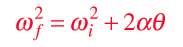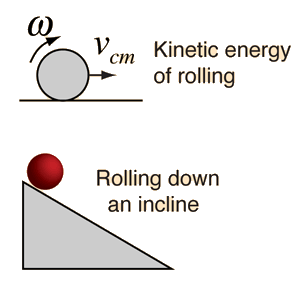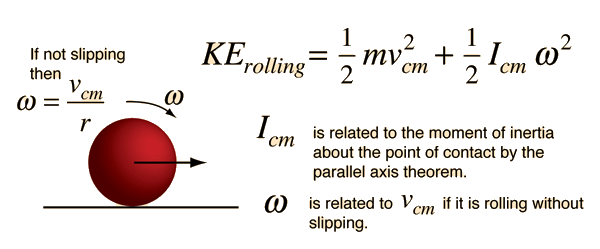# Work-Energy Principle

The work-energy principle is a general principle which can be applied specifically to rotating objects. For pure rotation, the net work is equal to the change in rotational kinetic energy:For a constant torque, the work can be expressed asand for a net torque, Newton's 2nd law for rotation givesCombining this last expression with the work-energy principle gives a useful relationship for describing rotational motion.Index

Rotation concepts

Rotational kinetic energy concepts

 HyperPhysics***** Mechanics ***** Rotation R Nave
Go Back

# Rolling ObjectsIn describing the motion of rolling objects, it must be kept in mind that the kinetic energy is divided between linear kinetic energy and rotational kinetic energy. Another key is that for rolling without slipping, the linear velocity of the center of mass is equal to the angular velocity times the radius.

### Hoop and cylinder on incline.

Index

Rotational kinetic energy concepts

 HyperPhysics***** Mechanics ***** Rotation R Nave
Go Back

# Kinetic Energy of Rolling Object

If an object is rolling without slipping, then its kinetic energy can be expressed as the sum of the translational kinetic energy of its center of mass plus the rotational kinetic energy about the center of mass. The angular velocity is of course related to the linear velocity of the center of mass, so the energy can be expressed in terms of either of them as the problem dictates, such as in the rolling of an object down an incline. Note that the moment of inertia used must be the moment of inertia about the center of mass. If it is known about some other axis, then the parallel axis theorem may be used to obtain the needed moment of inertia.Index

Rotation concepts

Rotational kinetic energy concepts

 HyperPhysics***** Mechanics ***** Rotation R Nave
Go Back